## Filters

Sort by :
Clear All
Q
Engineering
94 Views   |

The reverse biasing in a PN junction diode

• Option 1)

Decreases the potential barrier

• Option 2)

Increases the potential barrier

• Option 3)

Increases the number of minority charge carriers

• Option 4)

Increases the number of majority charge carriers

As we learnt in  P -N junction as diode - It is a one way device. It offers a low resistance when forward biased and high resistance when reverse biased. - wherein R = 0, Forward  R  Reverse    Do to reverse biasing potential barrier increases but it has no effect on number of charge carrier. Option 1) Decreases the potential barrier Incorrect Option 2) Increases the potential...
Engineering
139 Views   |

The approximate ratio of resistances in the forward and reverse bias of the PN-junction diode is

• Option 1)

• Option 2)

• Option 3)

• Option 4)

As we learnt in  P -N junction as diode - It is a one way device. It offers a low resistance when forward biased and high resistance when reverse biased. - wherein R = 0, Forward  R  Reverse    Ratio of forward to reverse bias resistance is of the order of  Option 1) Incorrect Option 2) Incorrect Option 3) Incorrect Option 4) Correct
Engineering
316 Views   |

PN-junction diode works as an insulator, if connected

• Option 1)

To A.C.

• Option 2)

In forward bias

• Option 3)

In reverse bias

• Option 4)

None of these

As we learnt in  P -N junction as diode - It is a one way device. It offers a low resistance when forward biased and high resistance when reverse biased. - wherein R = 0, Forward  R  Reverse   P N Junction in reverse biased mode offer infinitely large resistance and hence no current flow through it. Hence P N Junction in reverse bias act as an insulator. Option 1) To A.C.   Incorrect Option...
Engineering
133 Views   |

On increasing the reverse bias to a large value in a PN-junction diode, current.

• Option 1)

Increases slowly

• Option 2)

Remains fixed

• Option 3)

Suddenly increases

• Option 4)

Decreases slowly

As we learnt in  Zener diode - It can operate continuously without being damaged in the region of reverse  biased - wherein 1) It acts as voltage regulator 2) In forward biasing it act as ordinary diode .   On increasing reverse bias to a large value in a PN junction diode,current suddenly increase due to Zener / Avalanche breakdown. Option 1) Increases slowly   Incorrect Option 2) Remains...
Engineering
366 Views   |

Which of the following statements concerning the depletion zone of an unbiased PN junction is (are) true

• Option 1)

The width of the zone is independent of the densities of the dopants (impurities)

• Option 2)

The width of the zone is dependent on the densities of the dopants

• Option 3)

The electric field in the zone is produced by the ionized dopant atoms

• Option 4)

The electric field in the zone is provided by the electrons in the conduction band and the holes in the valence band

As learnt in P-N junction - When p type semiconductor is mixed with N - type semiconductor, P-N junction formed   - wherein There is very thin region (of the order of micro meter) called depution region.    Width of depletion region depend on densities of dopants. Both electron and holes are responsible for electric field in depletion zone. Option 1) The width of the zone is independent of...
Engineering
91 Views   |

When a PN junction diode is reverse biased

• Option 1)

Electrons and holes are attracted towards each other and move towards the depletion region

• Option 2)

Electrons and holes move away from the junction depletion region

• Option 3)

Height of the potential barrier decreases

• Option 4)

No change in the current takes place

Using P-N junction - When p type semiconductor is mixed with N - type semiconductor, P-N junction formed   - wherein There is very thin region (of the order of micro meter) called depution region.    and P -N junction as diode - It is a one way device. It offers a low resistance when forward biased and high resistance when reverse biased. - wherein R = 0, Forward  R  Reverse    When PN...
Engineering
92 Views   |

What is the current in the circuit shown below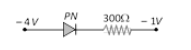• Option 1)

• Option 2)

• Option 3)

• Option 4)

As learnt in P -N junction as diode - It is a one way device. It offers a low resistance when forward biased and high resistance when reverse biased. - wherein R = 0, Forward  R  Reverse    The given diode is in reverse bias hence no current will flow through the resistor, I = 0 amp Option 1) This option is correct Option 2) This option is incorrect Option 3) This option is...
Engineering
250 Views   |

Two PN-junctions can be connected in series by three different methods as shown in the figure. If the potential difference in the junctions is the same, then the correct connections will be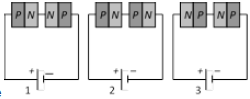• Option 1)

In the circuit (1) and (2)

• Option 2)

In the circuit (2) and (3)

• Option 3)

In the circuit (1) and (3)

• Option 4)

Only in the circuit (1)

As learnt in P -N junction as diode - It is a one way device. It offers a low resistance when forward biased and high resistance when reverse biased. - wherein R = 0, Forward  R  Reverse     In figure 2, both PN junction are forward biased and hence both will offer equal resistance and hence potential difference across the two are equal. In figure 3, both the PN junction are reverse biased...
Engineering
130 Views   |

The PN junction diode is used as

• Option 1)

An amplifier

• Option 2)

A rectifier

• Option 3)

An oscillator

• Option 4)

A modulator

Half Wave Rectifier - It is the type of rectification in which only one half of the input a.c. is translated into the output . - wherein Frequency of output is same as input a.c.     Full wave rectifier - It is type of rectification in which output is obtained for both the halves of a.c. - wherein Frequency of output is double to that of input    PN junction can be used as half wave or full...
Engineering
84 Views   |

The following truth table corresponds to the logic gate
A 0 0 1 1
B 0 1 0 1
X 0 1 1 0

• Option 1)

NAND

• Option 2)

OR

• Option 3)

AND

• Option 4)

XOR

OR gate - Relation between input and output      - wherein A and B are input Y is out put    X or gaH output =  Its truth table is    Option 1) NAND Incorrect option Option 2) OR Incorrect option Option 3) AND Incorrect option Option 4) XOR correct option
Engineering
526 Views   |

The electrical resistance of depletion layer is large because

• Option 1)

It has no charge carriers

• Option 2)

It has a large number of charge carriers

• Option 3)

It contains electrons as charge carriers

• Option 4)

It has holes as charge carriers

P-N junction - When p-type semiconductor is mixed with N-type semiconductor, P-N junction formed   - wherein There is a very thin region (of the order of the micrometre) called deputation region.    Since there is no hole or electron available in depletion region and hence it has a high electric resistance.   Option 1) It has no charge carriers Correct option Option 2) It has a large number...
Engineering
440 Views   |

The electrical circuit used to get smooth dc output from a rectifier circuit is called

• Option 1)

Oscillator

• Option 2)

Filter

• Option 3)

Amplifier

• Option 4)

Logic gates

Half Wave Rectifier - It is the type of rectification in which only one half of the input a.c. is translated into the output . - wherein Frequency of output is same as input a.c.     Full wave rectifier - It is type of rectification in which output is obtained for both the halves of a.c. - wherein Frequency of output is double to that of input    Filter is used to smoothen dc output from a...
Engineering
139 Views   |

The cut-in voltage for silicon diode is approximately

• Option 1)

0.2V

• Option 2)

0.6V

• Option 3)

1.1V

• Option 4)

1.4V

Knee voltage of P-N junction - It is defined as that forward voltage at which the current through the junction starts rising rapidly with increase in voltage . - wherein Knee voltage for Ge is 0.3 V Knee voltage for Si is 0.7 V   Cut in voltage for silicon diode is approximately 0.6V Option 1) 0.2V This solution is incorrect. Option 2) 0.6V This solution is correct. Option 3) 1.1V This...
Engineering
112 Views   |

In the forward bias arrangement of a PN-junction diode

• Option 1)

The N-end is connected to the positive terminal of the battery

• Option 2)

The P-end is connected to the positive terminal of the battery

• Option 3)

The direction of current is from N-end to P-end in the diode

• Option 4)

The P-end is connected to the negative terminal of battery

As we discussed in concept P -N junction as diode - It is a one way device. It offers a low resistance when forward biased and high resistance when reverse biased. - wherein R = 0, Forward  R  Reverse    In forward biased PN junction diode P type is connected to positive terminal of battery and N type with negative terminal of the battery. Option 1) The N-end is connected to the positive...
Engineering
101 Views   |

In the depletion region of an unbiased P-N junction diode there are

• Option 1)

Only electrons

• Option 2)

Only holes

• Option 3)

Both electrons and holes

• Option 4)

Only fixed ions

As we discussed in concept P-N junction - When p type semiconductor is mixed with N - type semiconductor, P-N junction formed   - wherein There is very thin region (of the order of micro meter) called depution region.    In the depletion region, all the holes and electrons combined together and anhiliate each other and hence only ions will be present in depletion region. Option 1) Only...
Engineering
186 Views   |

In the circuit given below, the value of the current is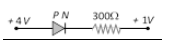• Option 1)

• Option 2)

• Option 3)

• Option 4)

As we discussed in concept P -N junction as diode - It is a one way device. It offers a low resistance when forward biased and high resistance when reverse biased. - wherein R = 0, Forward  R  Reverse    From Figure, potential difference = 3V. Since the diode is forward biased resistance offered by diode = 0 Option 1) This option is incorrect. Option 2) This option is correct. Option...
Engineering
99 Views   |

In the circuit below, identify the equivalent gate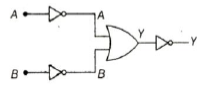• Option 1)

OR

• Option 2)

AND

• Option 3)

NOR

• Option 4)

NAND

As we learnt in  AND Gate - - wherein A and B are input Y is out put   Y=A . B This represent AND gate.   Option 1) OR This option is incorrect. Option 2) AND This option is correct. Option 3) NOR This option is incorrect. Option 4) NAND This option is incorrect.
Engineering
92 Views   |

In the circuit below, identify the equivalent gate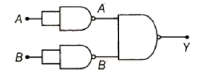• Option 1)

OR

• Option 2)

AND

• Option 3)

NOR

• Option 4)

NAND

OR gate - Relation between input and output      - wherein A and B are input Y is out put     D'morgan's Theorem - 1)     2)      3)     4)     - wherein A and B are input.     (D'morgabs law) This represent or gate   Option 1) OR Option is correct Option 2) AND Option is incorrect Option 3) NOR Option is incorrect Option 4) NAND Option is incorrect
Engineering
157 Views   |

In the circuit below, identify the equivalent gate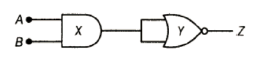• Option 1)

OR

• Option 2)

XOR

• Option 3)

NOT

• Option 4)

NAND

As we learnt in  NAND gate - NOT + AND gate - wherein A and B are input Y is out put     x= A.B Hence Final output   , represent NAND gate Option 1) OR Option is incorrect Option 2) XOR Option is incorrect Option 3) NOT Option is incorrect Option 4) NAND Option is correct
Engineering
134 Views   |

In forward bias, the width of potential barrier in a P-N junction diode

• Option 1)

Increases

• Option 2)

Decreases

• Option 3)

Remains constant

• Option 4)

First increases then decreases

P -N junction as diode - It is a one way device. It offers a low resistance when forward biased and high resistance when reverse biased. - wherein R = 0, Forward  R  Reverse   In forward bias, The width of potential barrier decreases and hence flow of current becomes high Option 1) Increases  Option is incorrect Option 2) Decreases Option is correct Option 3) Remains constant Option is...
Exams
Articles
Questions